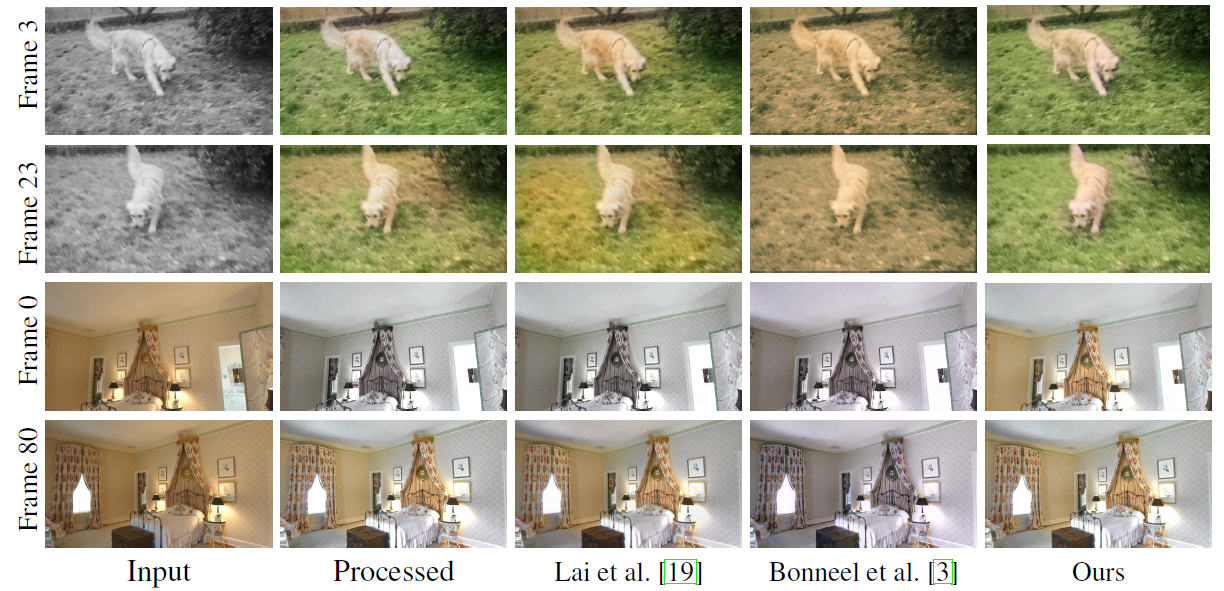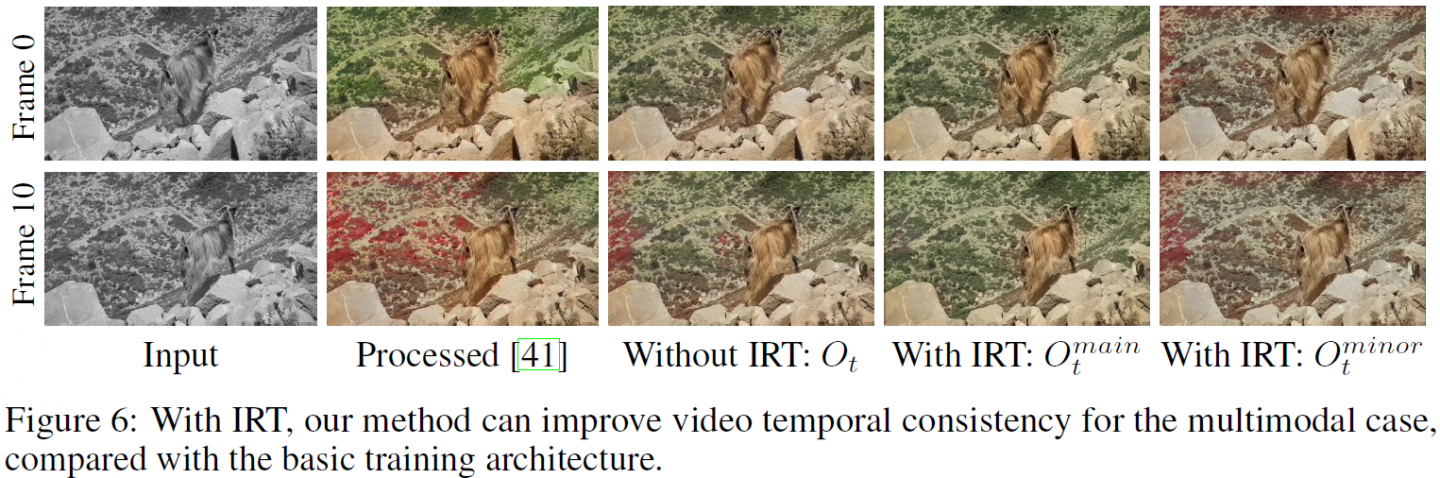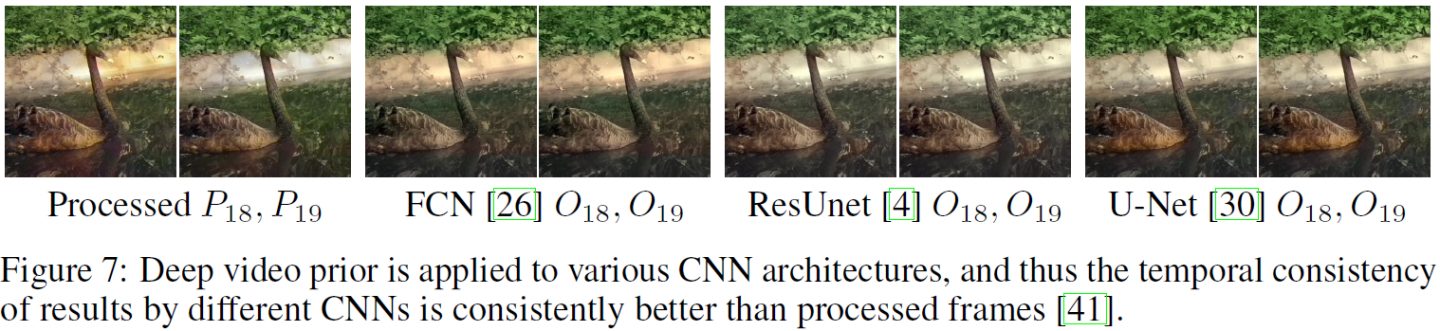## 图像算法处理视频不稳定？港科大团队提出一种通用算法解决视频处理时域不稳定问题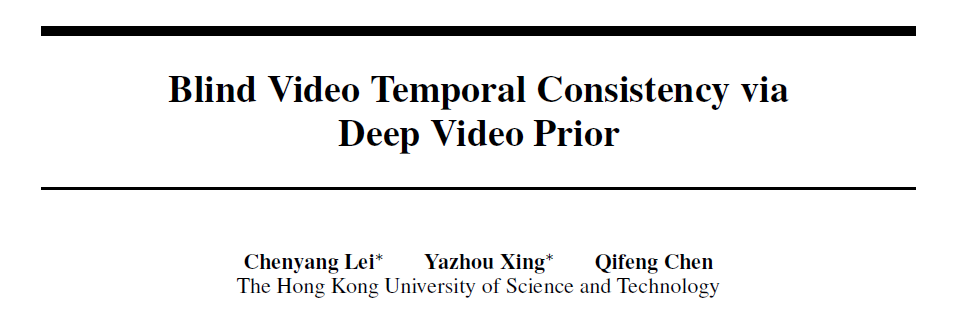arxiv链接：https://arxiv.org/abs/2010.11838

project主页：https://chenyanglei.github.io/DVP/index.html

video：https://youtu.be/07A3aRF4s0g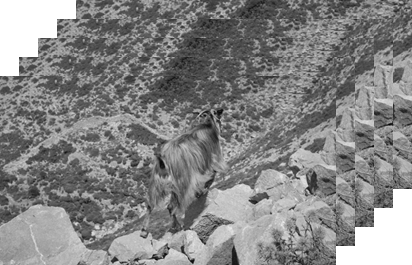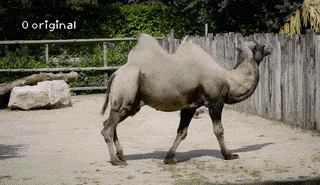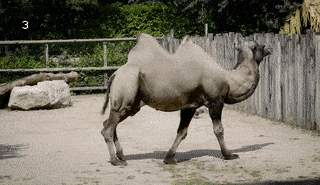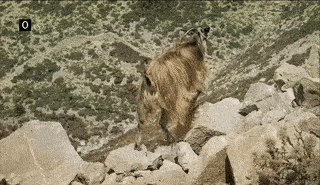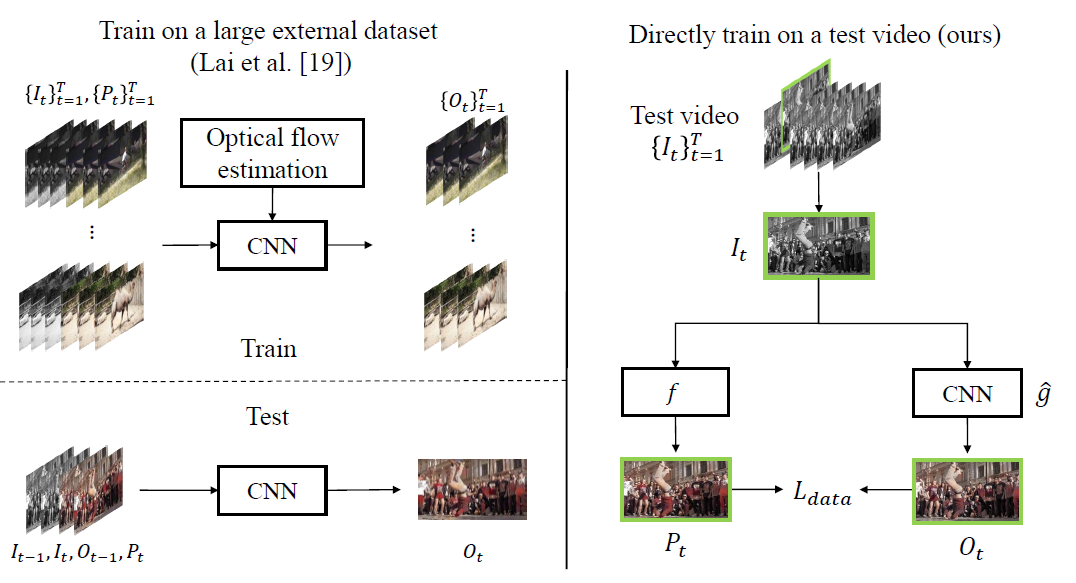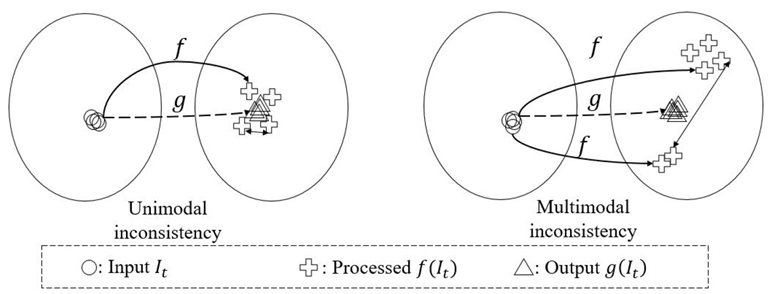θ i + 1 = arg ⁡ min ⁡ θ L _ data ( C _ t , i ⊙ O _ t , i main ⁡ , C _ t , i ⊙ P _ t ) + L _ data ( ( 1 − C _ t , i ) ⊙ O _ t , i minor ⁡ , ( 1 − C _ t , i ) ⊙ P _ t ) \begin{aligned} \theta^{i+1}=\underset{\theta}{\arg \min } & L\_{\text {data}}\left(C\_{t, i} \odot O\_{t, i}^{\operatorname{main}}, C\_{t, i} \odot P\_{t}\right)+\\ & L\_{\text {data}}\left(\left(1-C\_{t, i}\right) \odot O\_{t, i}^{\operatorname{minor}},\left(1-C\_{t, i}\right) \odot P\_{t}\right) \end{aligned}